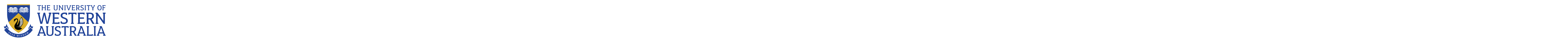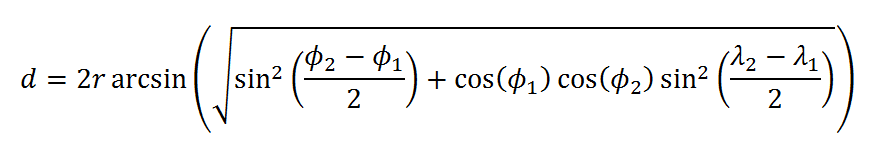CITS2002 Systems Programming

# Department of Computer Science and Software Engineering

## CITS2002 Systems Programming

### Workshop 3: Functions and parameter passing

In this workshop we'll develop a small number of functions to determine the distance between two points on the Earth's surface.

There are number of formulae that perform this calculation, some accounting for the fact that the Earth is not a true sphere.
One of the simplest is haversine's formula [Wikipedia]. We are interested in the simplified formula from Wikipedia:NOTE - this workshop is not requiring us to understand the formula, just to implement it in C99. Do not be alarmed by the use of spherical trigonometry (it won't be in the exam!)

The haversine function was used in the CIT2002 1st project from 2015.

Our goal is to develop 4 simple functions:

3. bool valid_location(double latitude, double longitude);

4. double haversine(double latitude1, double longitude1, double latitude2, double longitude2);

and to use them, in combination, to calculate the distance between the Computer Science building and the Tavern Science Library.

BEFORE the workshop session, you're strongly encouraged to think how you would do it. You are not expected to have developed and tested a perfect solution before the workshop, but you should at least scratch out your approach on paper.

From the information presented in lectures so far, we know:

• variables in our programs have a datatype which describes the meaning and (internal) representation of the variable's possible values.
• functions are named sequences of statements, that are either called to calculate a value, or to 'produce' a side-effect.
• functions receive zero or more parameters which provide input(s) to the function, or control its execution.
• like variables, function parameters also have a datatype (in fact, during the execution of each function, its parameters act like local variables).
• functions may return a single value, which also has a datatype, and this returned value may be used in further calculations, or printed...
Functions of type void return no value.

So, before the workshop session, think how you can combine this (already known) information to develop a solution.

• How can we TEST it?
• 🌶 For what values would the simpler formula $\sqrt{\mathrm{\Delta x2+ \Delta y2}}$ be accurate enough?

# The University of Western Australia

## University information

CRICOS Code: 00126G